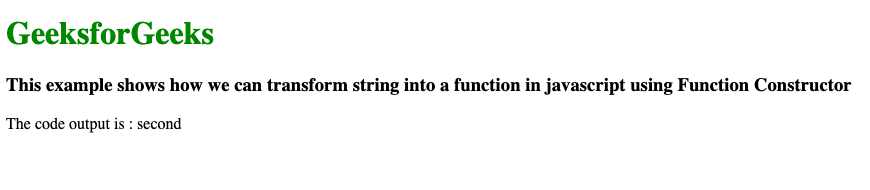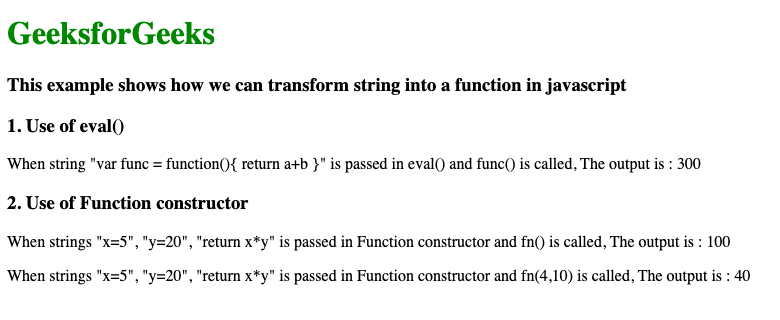# How to transform String into a function in JavaScript ?

• Last Updated : 26 Jun, 2022

In this article, we will see how to transform a String into a function in JavaScript. There are two ways to transform a String into a function in JavaScript. The first and easy one is eval() but it is not a secure method as it can run inside your application without permission hence more prone to third-party attacks. The second one is Function() which is way more secure than eval().

• Javascript eval(): This method takes a string as an argument and converts it into a function. Here the string can be a JavaScript expression, variable, statement, or sequence of statements.
• Function constructor: Functions created with the Function constructor do not create closures to their creation contexts; they are always created in the global scope. They will only be able to access their own local and global variables when they execute, not variables from the scope in which the Function constructor was created.

Example 1: The following code example shows another way to use Function Constructor to convert the string to function.

## HTML

 ` ` `<``html``> ` ` `  `<``body``> ` `    ``<``h1` `style``=``"color:green"``> ` `        ``GeeksforGeeks ` `    `` ` `     `  `    ``<``h3``> ` `        ``This example shows how we can transform string ` `        ``into a function in javascript using Function ` `        ``Constructor ` `    `` ` ` `  `    ``<``p``> The code output is : ` `        ``<``span` `id``=``"result"``> ` `    `` ` ` `  `    ``<``script``> ` `        ``var fnstring = "func"; ` `        ``var fnparams = ["first", "second", "third"]; ` ` `  `        ``// find an object ` `        ``var fn = window[fnstring]; ` ` `  `        ``// check if object a function? ` `        ``if (typeof fn === "function") { ` `            ``fn.apply(null, fnparams); ` `        ``} ` ` `  `        ``function func(a, b, c) { ` `            ``document.getElementById("result").innerHTML = b; ` `        ``} ` `    `` ` ` ` ` `  ` `

Output:Example 2: The below code example demonstrate different ways to convert a string into a function.

## HTML

 ` ` `<``html``> ` ` `  `<``body``> ` `    ``<``h1` `style``=``"color:green;"``> ` `        ``GeeksforGeeks ` `    `` ` `     `  `    ``<``h3``> ` `        ``This example shows how we can transform string ` `        ``into a function in javascript ` `    `` ` `    ``<``h3``>1. Use of eval() ` ` `  `    ``<``p``> ` `        ``When string "var func = function(){ return a+b }" is ` `        ``passed in eval() and func() is called, The output is : ` `        ``<``span` `id``=``"result"``> ` `    `` ` ` `  `    ``<``h3``>2. Use of Function constructor ` ` `  `    ``<``p``> ` `        ``When strings "x=5", "y=20", "return x*y" is ` `        ``passed in Function constructor and fn() is called, ` `        ``The output is : ` `        ``<``span` `id``=``"func1"``> ` `    `` ` ` `  `    ``<``p``> ` `        ``When strings "x=5", "y=20", "return x*y" is ` `        ``passed in Function constructor and fn(4,10) is called, ` `        ``The output is : ` `        ``<``span` `id``=``"func2"``> ` `    `` ` ` `  `    ``<``script``> ` `        ``// use of eval()     ` `        ``var a = 100; ` `        ``var b = 200; ` `        ``eval("var func = function(){ return a+b }"); ` `        ``document.getElementById("result").innerHTML = func(); ` `        ``// Use of Function Constructor ` `        ``const fn = new Function("x=5", "y=20", "return x*y"); ` `        ``document.getElementById("func1").innerHTML = fn(); ` `        ``document.getElementById("func2").innerHTML = fn(4, 10); ` `    `` ` ` ` ` `  ` `

Output: The output shows the transformation of a string into a function.My Personal Notes arrow_drop_up
Recommended Articles
Page :